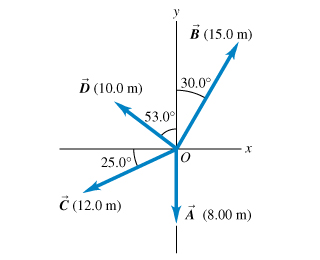# Problem: Write each vector in the figure in terms of the unit vectors i and j.

###### FREE Expert Solution

2D vector Components:

Vector A has only x-component.

A = 8.00m i + 0j

The expression for vector A in terms of the unit vectors i and j is 8.00m i + 0j

98% (367 ratings)###### Problem Details

Write each vector in the figure in terms of the unit vectors i and j.